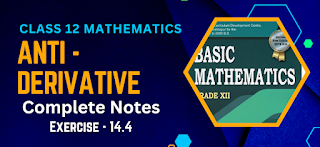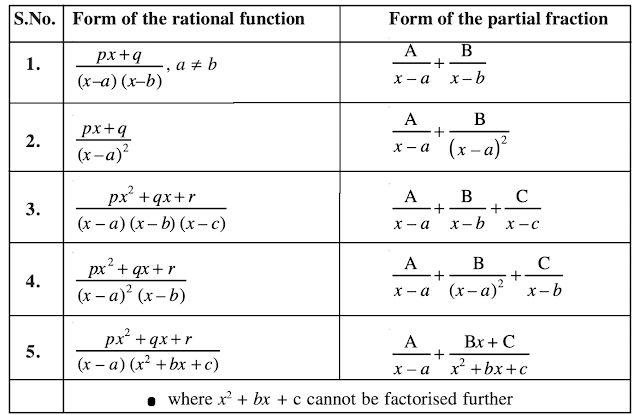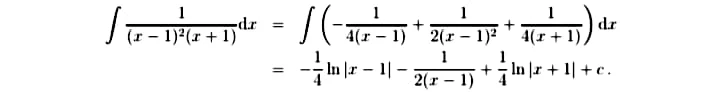# Antiderivative Notes Class 12 Mathematics | Exercise - 14.4Antiderivative Notes Class 12 Mathematics as per the latest syllabus of 2080. If you ate struggling to solve the questions of antiderivative then you can take help from antiderivatives notes class 12 mathematics. Scroll down to get complete solution of class 12 antiderivatives chapter fourth exercise. You can also download the notes PDF so you can view them even if you are offline.

## Chapter - 14  Antiderivative

There are total four exercises in class 12 mathematics antiderivative chapter.  Till now, we have discussed many topics in first, second and third exercises. We have also discussed the exercises of all previous exercises. Now, here in this exercise we'll be discussing some new topics.

This exercise is totally based on integration of rational fractions. It includes all the formulas,  methods of finding integration by rational fraction method and many more. So let's learn about it in brief.

### Integration of Rational Fractions

The integration of an algebraic rational fraction is generally not possible as it is. To make its integration possible, the rational function must be resolved into partial fractions.

Often, the denominator of the given rational fraction can be resolved into real linear and quadratic factors which may be single or repeated.

According to the nature of the factors of the denominator, we have to adopt different procedures for the breaking up of the rational fraction into partial fractions.

To some extent, we have given some simple methods of breaking some algebraic rational fractions into partial fractions. Now we use this knowledge to integrate some algebraic rational fractions.

Exercise - 14.4

This PDF contains 15 question which are extracted from the fourth exercise of antiderivative chapter of class 12. All the solutions are in handwritten format. If you want the notes of previous exercise then you can click the buttons given above.

Note: Scroll the PDF to view all solutions

#### Formula used in Integration of Rational Fractions

In integration of rational fractions class 12, we will be using some important formula that will help us to find the integration of the given questions. These formulas may be new for grade 12 students as there were no such formulas in class 11 antiderivative chapter.

Have a look at the formulas before sokvthe questions of the fourth exercise. The formulas may seems complicated to remember but they are super easy once you understand it.#### Some Examples of Integration by Partial Fractions

Find ∫ 1/ [(x - 1)² (x + 1)]dx
In this Example there is a repeated factor in the denominator. This is because the factor (x-1) apears twice, as in (x − 1)². We write

1/(x - 1)²(x + 1) = A / (x - 1) + B / (x -1)² + C/(x+1)
= {A(x - 1)(x + 1) + B(x + 1) + C(x - 1)²}/(x - 1)²(x + 1)

As before, the fractions on the left and the right are equal for all values of x. Their denominators are equal and so we can equate the numerators:

1 = A(x - 1)(x + 1) + B(x + 1) + C(x - 1)²

Substituting x = 1 in Equation (1) gives 1 = 2B, from which B = 1/2.
Substituting x = −1 gives 1 = 4C from which C = 1/4.

Knowing B and C, substitution of any other value for x will give the value of A. For example, if we let x = 0 we find

1 = −A + B + C And also,
1 = −A + 1/2 + 1/4

from which A = -1/4. Alternatively, we could have expanded the right-hand side of Equation (1), collected like terms together and equated coefficients. This would have yielded the same values for A, B and C.

The integral becomes;## Some Important Questions

Now we have finished discussing about the main topics including in the exercise, it's time to learn some of the important questions that might get asked as a 1 mark question in your examination. We have already discussed more than 10 questions in our previous exercises and in this exercise we will be discussing some more questions.

### 1. How do you integrate fractions?

To integrate fractions, you can use a method called partial fraction decomposition. Here's a general approach:

• Factorize the denominator of the fraction into irreducible factors.
• Now express the fraction as a sum of simpler fractions, where each denominator is one of the irreducible factors.
• Determine the unknown coefficients for each fraction by comparing the numerators on both sides of the equation.
• Integrate each fraction separately using standard integration techniques.
• Sum up the integrated fractions to obtain the final result.

You have to keep in mind that this method is used when the degree of the numerator is less than the degree of the denominator. If not, you may need to use other integration techniques like polynomial long division or trigonometric substitutions.

### 2. How do you integrate fractions with trigonometry?

If you have to integrate  fractions with trigonometric functions then you can use a method called trigonometric substitution. To use this method, you have to substitute the expression under the square root with a trigonometric function, simplify the resulting expression using trigonometric identities, integrate it, and then substitute back the original trigonometric function to obtain the final result.

### 3. What is integration for sin and cos?

The integration of sine and cosine functions involves evaluating antiderivatives. Here are the integration formulas for sin(x) and cos(x):

∫sin(x) dx = -cos(x) + C
∫cos(x) dx = sin(x) + C

We have discussed about these formulas in previous exercises. This formula represent the antiderivatives of sine and cosine functions, where C is the constant of integration. By applying these formulas, you can find the integral of a given sine or cosine function.

No Comment